## Chapter 2Fluid Dynamics

“Stands on shifting sands, the scales held in her hands. The wind it just whips her and wails and ﬁlls up her brigantine sails.”

The Stone Roses, Waterfall (1989).

This chapter introduces ﬂuid dynamics for CFD. It describes: governing equations, i.e. conservation of mass, momentum and energy; and, associated physical models, e.g. for viscosity, heat conduction and thermodynamics.

The equations describe ﬂuid motion, forces and heat in time and three-dimensional (3D) space. Vector notation provides a mathematical framework to present the equations in a compact form. It enables the equations to be presented independently of any co-ordinate system, e.g. Cartesian (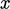/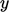/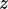) or spherical (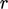/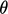/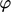). It includes a standard set of algebraic operations, e.g. the inner (dot) and outer products.

The notation helps to ensure that the terms in equations are unchanged, or invariant, under a co-ordinate system transformation. Without invariance, a ﬂow solution, e.g. along a pipe, would be dependent on the orientation of the pipe with respect to the co-ordinate system. Logically this dependence cannot exist; the laws of motion are the same in all “inertial frames”.1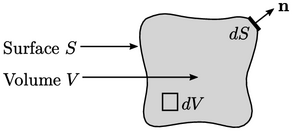The derivation of the governing equations uses a control volume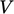bounded by a surface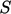, presented using the two-dimensional (2D) illustration above. We use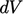and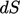to describe an inﬁnitesimally small volume and surface, respectively, and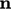is the unit normal vector for each increment of surface, discussed in Sec. 2.1 . It is important to note in any derivation whether the volume is deﬁned as ﬁxed in space or moving with the ﬂuid.

Each derivation generally begins with a deﬁnite integral of some quantity, e.g.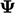, over the volume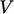denoted by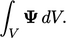(2.1)
If this notation is unfamiliar, understand it to mean a summation for all increments of volumethat make up the total volume. The summed values are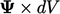, where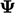is the value in the respective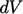.

The derivations also use integrals over the surface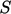, e.g.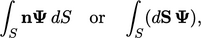(2.2)
where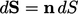. Volume and surface integrals are connected through Gauss’s Theorem, introduced in Sec. 2.4 .
1Galileo Galilei, Dialogo sopra i due massimi sistemi del mondo, 1632.

2.1 Pressure
2.2 Velocity

2.11 Vorticity
2.12 Newtonian ﬂuid

2.14 Diﬀusion

2.16 Temperature
2.17 Internal energy
2.18 Heat capacity

2.21 Scale similarity

Notes on CFD: General Principles - Chapter 2 Fluid Dynamics